# Introduction to the package ecochange

## Introduction

The R-package ecochange (Lara et al., 2022) can assist users in monitoring ecosystem changes by computing indicators related to essential biodiversity variables in the class ecosystem structure (Pereira et al., 2013), including ecosystem extent, horizontal structure, and ecosystem fragmentation.

The package requires minimally the name of an administrative unit or a user-defined polygon and the name of a remote sensing product. The names of administrative units come from Geographic Administrative Data Maps (GADM). Remote sensing products consist of 16 different data layers that can be downloaded using the package functionality from three global databases: Forest Cover (GFC; Hansen et al., 2013), Tree-canopy Cover (TC; Sexton et al., 2013), and Water Surface (GWS; Pekel et al., 2017). The package can also process other custom-based datasets.

ecochange integrates all remote sensing products to the geometry of the polygon. Then it combines the datasets to produce a variety of spatial products, indicators, statistics and graphs. Most arguments are inherited by different functions to make the coding more efficient.

## Installation

Users can install the package and the dependencies running install.packages(). They can load it using either require() or library(). We recommend users install and load the package viridis. this package improves the color palettes of the plots.

# install.packages('ecochange')

require('ecochange')
require('viridis')

The function getrsp() downloads a data tile for a selected RS product covering a polygon. Its first argument can either be the name of an administrative unit or a polygon.

# The getGADM() function provides the names of administrative units. By default, it shows level 2 administrative units in the country of Colombia but those settings can be changed through the parameters level and country

listGP()

# of Chimichagua
waterocc=getrsp("Chimichagua", lyrs=c("occurrence"))

# Users can define a different path using the argument path()
waterocc

# Let's plot the object
plot(raster(waterocc), axes = T,
main = 'Occurrence (Colombia)')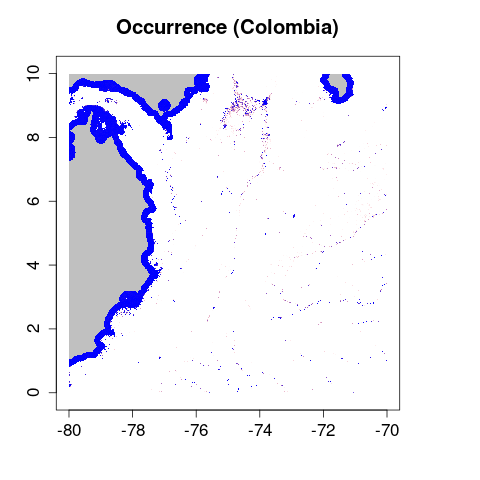wo=rsp2ebv('Chimichagua', lyrs = c('occurrence'), mc.cores = detectCores())
plot(wo, main = 'Occurrence (Municipality of Chimichagua)')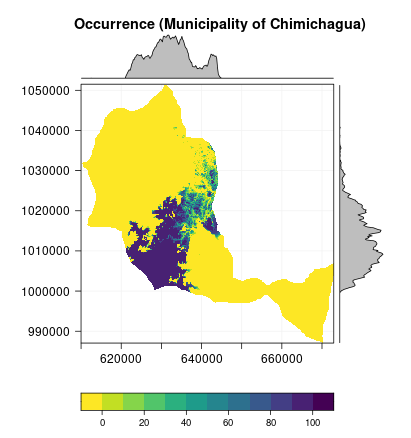# check that the projection is different to the file that was originally downloaded
crs(raster(waterocc))
crs(wo[])

The rsp2ebv() can also be implemented to download and integrate several datasets simultaneously.

ebv <- rsp2ebv('Chimichagua', lyrs = c('treecover2000','lossyear'), mc.cores = detectCores())
plot(ebv, main = 'Forest cover and loss (Chimichagua)')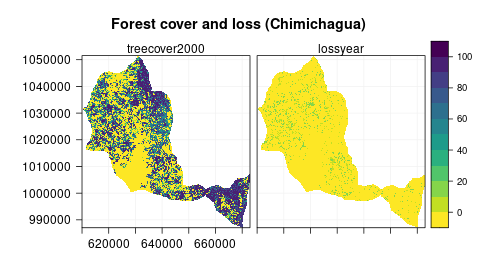## Deriving ecological changes

Function echanges() can produce RasterStack ecosystem-change representations by masking cell values in a layer of ecosystem changes over a target ecosystem variable.

This function can process datasets previously integrated with rsp2ebv(). It can also inherit arguments in rsp2ebv() and derive the data sections as an intermediate step.

The function is designed to be generic so that it can adapt to broad types of datasets and purposes. By default, echanges() considers the first layer as the target variable and the last layer as the change raster. If these layer positions are incorrect, the users must provide two additional arguments: eco and change. Such arguments provide regular expressions matching the names of the variables in the raster-data sections. For instance, we can use the arguments eco and change to provide the expressions ‘tree’ and ‘loss’ corresponding with the layers ‘treecover2000’ and ‘lossyear’, respectively.

Arguments eco_range and change_vals control the pixel values processed in the target variable and the change raster.

The output of echanges() is a RasterStack object with dimensionality defined by the values provided in change_vals. Here we obtain three layers corresponding with deforestations observed during the three years.

For instance, let’s calculate forest cover area for the years 2005, 2010, and 2020, assuming a definition of forest as those areas with canopy cover between 95 and 100%

forExt <- echanges(ebv, eco = 'tree', eco_range=c(95,100), echanges = 'loss', change_vals = c(5,10,20), binary_output=TRUE, mc.cores = detectCores())
plot(forExt, main = 'Changes in forest cover')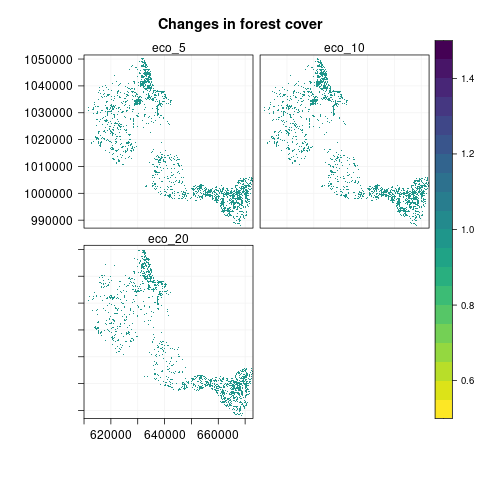It is possible to produce instead, a map with cumulative deforested areas by setting the argument get_unaffected=FALSE.

defExt <- echanges(ebv, eco = 'tree', eco_range=c(95,100), echanges = 'loss', change_vals = c(5,10,20), binary_output=TRUE, mc.cores = detectCores(),
get_unaffected=FALSE)
plot(defExt, main = 'Changes in forest loss')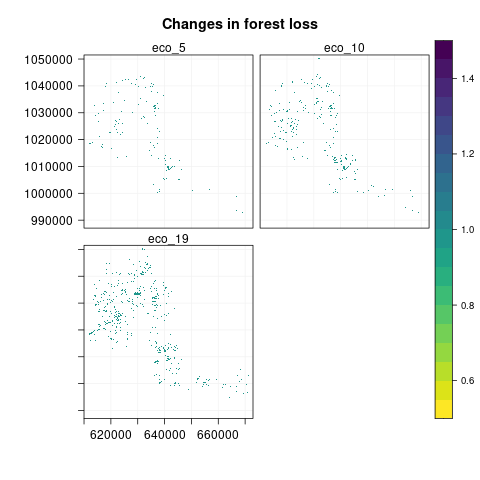The function also allows to calculate, for instance, the distribution of tree cover among deforested pixels by changing the argument binary_output = FALSE.

defExtTC <- echanges(ebv, eco = 'tree', eco_range=c(95,100), echanges = 'loss', change_vals = c(5,10,20), binary_output=FALSE, mc.cores = detectCores(), get_unaffected=FALSE)
plot(defExtTC, main = 'Changes in deforested pixels')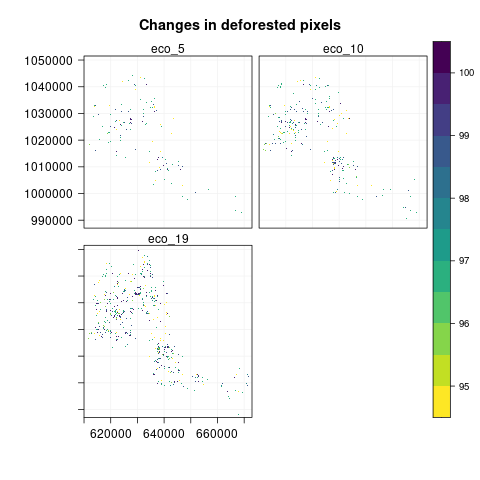## Calculating biodiversity indicators and statistics

The function gaugeIndicator() calculates biodiversity indicators by processing RasterStack ecosystem-change representations from echanges(). It can also inherit arguments from rsp2ebv() and echanges() to derive the RasterStack ecosystem-change representations.

The argument metric provides the name of a biodiversity indicator. By default, metric = ‘area_ha’ tells the function to compute ecosystem areas (hectare).

Users can define any other biodiversity indicators inherited from the package landscapemetrics (Hesselbarth et al., 2019)

library(landscapemetrics)
list_lsm()

The function output is a tibble of indicators ordered according to classes in the ecological variable. This output can be visualized using the in-package plot.Indicator().

Let’s calculate the cumulative forest and deforested area in the three years analyzed above

forArea=gaugeIndicator(forExt, ncores=6)
defArea=gaugeIndicator(defExt, ncores=6)
forArea
defArea
plot(forArea, cex = 1.1, xlab = 'Year', ylab = 'Area (ha)', title = "Ecosystem extents", subtitle = 'Forest cover', fill = 'Pixel \nvalue')
plot(defArea, cex = 1.1, xlab = 'Year', ylab = '', title = '', subtitle = 'Forest loss', fill = '')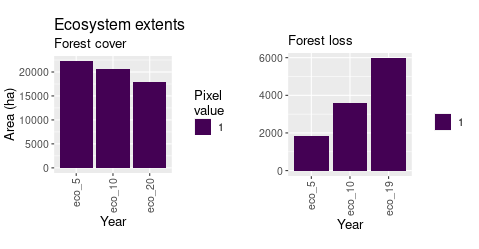The function allows to calculate the area of forest loss per canopy cover classes. We adjust the color palette in the plot.Indicator method to better visualize the area covered per class

defAreaTC=gaugeIndicator(defExtTC, ncores=6)
defAreaTC
plot(defAreaTC, y = viridis(6), cex = 1.1, xlab = 'Year',
ylab = 'Area (ha)', title = 'Forest loss',
subtitle = 'Tree-canopy cover values', fill = '(%)')

Users can reproduce box plots over time by setting type = ‘b’ in the very same plot method

plot(defAreaTC, type = 'b', cex = 1.1, xlab = 'Year',
ylab = '',title = '', subtitle = 'Tree-canopy cover distributions', fill = 'Year')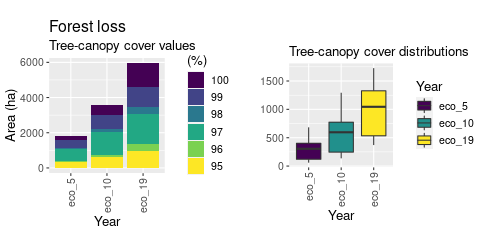Users can also calculate summary statistics for each tree cover class using the EBVstats() function:

chgstats=EBVstats(defExtTC)
chgstats

## Sampling indicators across grids

The function sampleIndicator() divides each of the scenes in a stack into fixed-size grids and produces a biodiversity indicator for each grid. This function helps users to visualize changes in the spatial distribution of the biodiversity indicators.

Here we calculate changes in conditional entropy to measure ecosystem degradation in terms of changes of pixel adjacency and canopy-cover diversity. The function includes an argument side that defines the cell-size. Users can use this argument to define a customized grid size. Using the default settings for the side argument means that the algorithm will automatically produce an optimum grid size (m) that returns at least a numeric value for each grid in the scene. The function output is a RasterStack object with the same number of layers as the ecosystem-change map.

# Let's first calculate forest extent by all tree cover classes for 2019
ech <- echanges(ebv, eco = 'tree', echanges = 'loss',
change_vals = c(0,20), mc.cores = 2)
plot(ech, main = 'Forest cover')

si <- sampleIndicator(ech, mc.cores = detectCores())
si
plot(si, main = 'Conditional entropy')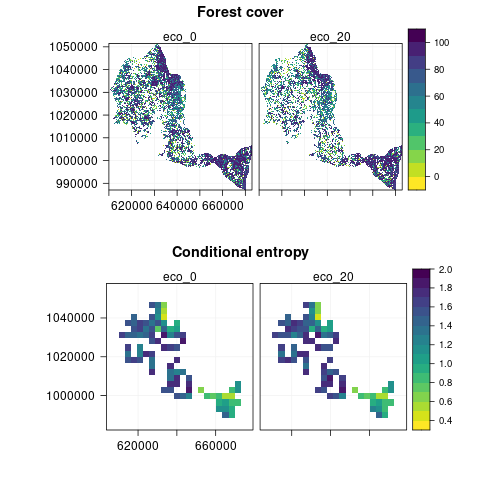## Citing ecochange

The package can be cited using the citation() function. Please cite ecochange appropriately in your work.

citation('ecochange')

## Data sources and references

Hansen, M. C., Potapov, P. V., Moore, R., Hancher, M., Turubanova, S. A. A., Tyukavina, A., … & Kommareddy, A. (2013). High-resolution global maps of 21st-century forest cover change. science, 342(6160), 850-853.

Hesselbarth, M.H., Sciaini, M., With, K.A., Wiegand, K., & Nowosad, J., (2019). landscapemetrics: an open-source R tool to calculate landscape metrics. Ecography.

Lara, W., Londoño, M. C., Gonzalez, I., & Gutierrez‐Velez, V. H. (2022). ecochange: An R‐package to derive ecosystem change indicators from freely available earth observation products. Methods in Ecology and Evolution, 13(11), 2379-2388.

Pekel, J. F., Belward, A., & Gorelick, N. (2017). Global Water Surface Dynamics: Toward a Near Real Time Monitoring Using Landsat and Sentinel Data. In AGU Fall Meeting Abstracts.

Pereira, H.M., Ferrier, S., Walters, M., Geller, G.N., Jongman, R.H.G., Scholes, R.J., Bruford, M.W., Brummitt, N., Butchart, S.H.M., Cardoso, A.C. and Coops, N.C., 2013. Essential biodiversity variables. Science, 339(6117), pp.277-278.

Sexton, J. O., Song, X. P., Feng, M., Noojipady, P., Anand, A., Huang, C., … & Townshend, J. R. (2013). Global, 30-m resolution continuous fields of tree cover: Landsat-based rescaling of MODIS vegetation continuous fields with lidar-based estimates of error. International Journal of Digital Earth, 6(5), 427-448.